# Edge coloring

## Petersen coloring conjecture ★★★

Author(s): Jaeger

Conjecture   Letbe a cubic graph with no bridge. Then there is a coloring of the edges ofusing the edges of the Petersen graph so that any three mutually adjacent edges ofmap to three mutually adjancent edges in the Petersen graph.

Keywords: cubic; edge-coloring; Petersen graph

## Packing T-joins ★★

Author(s): DeVos

Conjecture   There exists a fixed constant(probably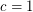suffices) so that every graft with minimum-cut size at leastcontains a-join packing of size at least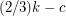.

Keywords: packing; T-join

## Acyclic edge-colouring ★★

Author(s): Fiamcik

Conjecture   Every simple graph with maximum degreehas a proper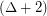-edge-colouring so that every cycle contains edges of at least three distinct colours.

Keywords: edge-coloring

## A generalization of Vizing's Theorem? ★★

Author(s): Rosenfeld

Conjecture   Letbe a simple-uniform hypergraph, and assume that every set of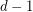points is contained in at mostedges. Then there exists an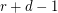-edge-coloring so that any two edges which sharevertices have distinct colors.

Keywords: edge-coloring; hypergraph; Vizing

## List colorings of edge-critical graphs ★★

Author(s): Mohar

Conjecture   Suppose thatis a-edge-critical graph. Suppose that for each edgeof, there is a listofcolors. Thenis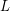-edge-colorable unless all lists are equal to each other.

Keywords: edge-coloring; list coloring

## Universal Steiner triple systems ★★

Author(s): Grannell; Griggs; Knor; Skoviera

Problem   Which Steiner triple systems are universal?

Keywords: cubic graph; Steiner triple system

## Edge list coloring conjecture ★★★

Author(s):

Conjecture   Letbe a loopless multigraph. Then the edge chromatic number ofequals the list edge chromatic number of.

Keywords:

## Seymour's r-graph conjecture ★★★

Author(s): Seymour

An-graph is an-regular graphwith the property that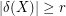for every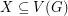with odd size.

Conjecture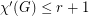for every-graph.

Keywords: edge-coloring; r-graph

## Goldberg's conjecture ★★★

Author(s): Goldberg

The overfull parameter is defined as follows:Conjecture   Every graphsatisfies.

Keywords: edge-coloring; multigraph

## Strong edge colouring conjecture ★★

Author(s): Erdos; Nesetril

A strong edge-colouring of a graphis a edge-colouring in which every colour class is an induced matching; that is, any two vertices belonging to distinct edges with the same colour are not adjacent. The strong chromatic index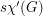is the minimum number of colours in a strong edge-colouring of.

ConjectureKeywords: